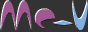Infinite MathsStart: 30th May 6:10

This site has been spun off on it's own, at 0maths.com

Welcome to infinite maths: a bottomless pit of rapid fire, randomly generated practice questions across 37 topics including addition, subtraction, division, multiplication, percentages, units, fractions, factors, time, money, units, geometry, area and algebra, available free, without sign up. The more you answer, the harder they get.

There are no wrong answers, only answers that aren't yet right. There's no submit button - you get the next question when you get the answer right. If you really can't get it, press skip. In a lot of cases, particularly where technique is not intuitive, the skipped question becomes a worked example. A similar question is then given.

Show me the maths!

The difficulty level will (by default) increase with every question answered correctly. Alternatively, you can use the difficulty slider at the top of the topics, and it will give you a note, by each topic to explain what makes it harder or easier.

Topics covered include:
• Addition to a set number
• Subtraction to a set number
• Find the missing number to make ten, twenty, or a hundred
• Decimals
• Money
• Multiplication & division
• Multiplication with selected times table(s)
• Division with selected times table(s)
• Find the factors
• Highest Common Factor
• Money - using British notes and coins
• Counting money
• Make a total
• Give the right change
• Time & speed
• Telling the time
• Roman Numerals
• Speed & distance
• Units
• Convert between micro, milli, centi, deci, kilo, etc
• Fractions and other unwholey numbers
• Simplify fractions
• Top heavy fractions
• Fractions to decimals
• Fractions from decimals
• Subtraction
• Multiplication
• Division
• Fractions to percentages and decimals and back again
• Rounding decimals
• Geometry
• Rectangles -area & perimeter
• Circles -area & circumference
• Triangles - area
• Pythagoras - sides & perimeter
• Sine, Cosine & Tangent
• Algebra
• Simplify expressions
• Calculate x
• Expand brackets
• Bidmas
• Statistics
• Averages - mean, median & mode
• Probability
Select Topic(s)

Difficulty: 1  Right:0 Skipped: 0 Level: